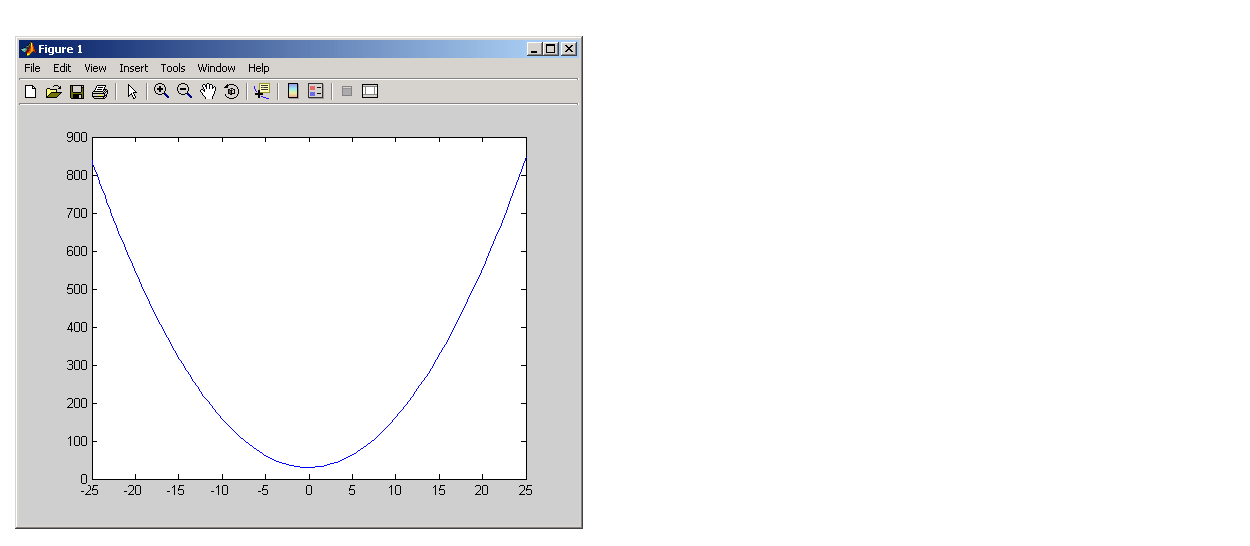ProgrammingExamples of Nested Functions

This section shows a few examples of how you can use nested functions. These examples are intended to show you how to program with this type of function. For more mathematically oriented examples, see the MATLAB Mathematics documentation.

The examples in this section include

Example 1 -- Creating a Function Handle for a Nested Function

The following example constructs a function handle for a nested function and then passes the handle to the MATLAB `fplot` function to plot the parabola shape. The `makeParabola` function shown here constructs and returns a function handle `fhandle` for the nested `parabola` function. This handle gets passed to `fplot`:

• ```function fhandle = makeParabola(a, b, c)
% MAKEPARABOLA returns a function handle with parabola
% coefficients.

fhandle = @parabola;       % @ is the function handle constructor

function y = parabola(x)
y = a*x.^2 + b*x + c;
end
end
```

Assign the function handle returned from the call to a variable (`h`) and evaluate the function at points 0 and 25:

• ```h = makeParabola(1.3, .2, 30)
h =
@makeParabola/parabola

h(0)
ans =
30

h(25)
ans =
847.5000
```

Now pass the function handle `h` to the `fplot` function, evaluating the parabolic equation from `x = -25` to `x = +25`:

• ```fplot(h, [-25 25])```

Example 2 -- Function-Generating Functions

The fact that a function handle separately maintains a unique instance of the function from which it is constructed means that you can generate multiple handles for a function, each operating independently from the others. The function in this example makes IIR filtering functions by constructing function handles from nested functions. Each of these handles maintains its own internal state independent of the others.

The function `makeFilter` takes IIR filter coefficient vectors `a` and `b` and returns a filtering function in the form of a function handle. Each time a new input value `x`n is available, you can call the filtering function to get the new output value `y`n. Each filtering function created by `makeFilter` keeps its own private `a` and `b` vectors, in addition to its own private state vector, in the form of a transposed direct form II delay line:

• ```function [filtfcn, statefcn] = makeFilter(b, a)
%   FILTFCN = MAKEFILTER(B, A) creates an IIR filtering
%   function and returns it in the form of a function handle,
%   FILTFCN. Each time you call FILTFCN with a new filter
%   input value, it computes the corresponding new filter
%   output value, updating its internal state vector at the
%   same time.
%
%   [FILTFCN, STATEFCN] = MAKEFILTER(B, A) also returns a
%   function (in the form of a function handle, STATEFCN)
%   that can return the filter's internal state.  The internal
%   state vector is in the form of a transposed direct form
%   II delay line.

%   Initialize state vector. To keep this example a bit simpler,
%   assume that a and b have the same length.  Also assume that
%   a(1) is 1.

v = zeros(size(a));

filtfcn =  @iirFilter;
statefcn = @getState;

function yn = iirFilter(xn)
% Update the state vector
v(1) = v(2) + b(1) * xn;
v(2:end-1) = v(3:end) + b(2:end-1) * xn - a(2:end-1) * v(1);
v(end) = b(end) * xn - a(end) * v(1);

% Output is the first element of the state vector.
yn = v(1);
end

function vOut = getState
vOut = v;
end
end
```

This sample session shows how `makeFilter` works. Make a filter that has a decaying exponential impulse response and then call it a few times in succession to see the output values change:

• ```[filt1, state1] = makeFilter([1 0], [1 -.5]);

% First input to the filter is 1.
filt1(1)
ans =
1

% Second input to the filter is 0.
filt1(0)
ans =
0.5000

filt1(0)
ans =
0.2500

% Show the filter's internal state.
state1()
ans =
0.2500    0.1250

% Hit the filter with another impulse.
filt1(1)
ans =
1.1250

% How did the state change?
state1()
ans =
1.1250    0.5625

% Make an averaging filter.
filt2 = makeFilter([1 1 1]/3, [1 0 0]);

% Put a step input into filt2.
filt2(1)
ans =
0.3333

filt2(1)
ans =
0.6667

filt2(1)
ans =
1

% The two filter functions can be used independently.
filt1(0)
ans =
0.5625
```

As an extension of this example, suppose you were looking for a way to develop simulations of different filtering structures and compare them. This might be useful if you were interested in obtaining the range of values taken on by elements of the state vector, and how those values compare with a different filter structure. Here is one way you could capture the filter state at each step and save it for later analysis:

Call `makeFilter` with inputs `v1` and `v2` to construct function handles to the `iirFilter` and `getState` subfunctions:

• ```[filtfcn, statefcn] = makeFilter(v1, v2);
```

Call the `iirFilter` and `getState` functions by means of their handles, passing in random values:

• ```x = rand(1, 20);
for k = 1:20
y(k) = filtfcn(x(k));
states{k} = statefcn();  % Save the state at each step.
end
```Using Function Handles with Nested Functions Subfunctions© 1994-2005 The MathWorks, Inc.